# 线性调频信号

### 公式推导

线性调频信号的数学表达式：其中，t是时间变量，单位为秒（s）；T为脉冲持续时间（周期）；K是线性调频率，单位是Hz/s；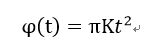对时间取微分后的瞬时频率为：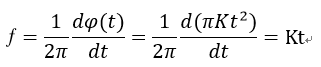信号的带宽是Chrip信号的斜率和时间的乘积：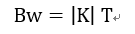带宽决定了能够达到的分辨率。

Matlab代码如下：

close all;clc;clear all;
%%
%   LFM ：linear frequency modulation
%%
B = 70e6; % 带宽70MHz
T = 2e-6; % 脉宽20us
Fs = 100e6; % 采样率
N = T*Fs;
t = -T/2:1/Fs:T/2-1/Fs;
K = B/T;
%%
St = exp(j*pi*K*t.^2); %信号
theta = pi*K*t.^2; %信号弧度
f = K*t; %信号频率

figure
subplot(2,2,1);plot(real(St));title('信号实部');
subplot(2,2,2);plot(imag(St));title('信号虚部');
subplot(2,2,3);plot(theta);title('信号相位 °');
subplot(2,2,4);plot(f);title('信号频率 Hz');

figure;
plot(abs(fftshift(fft(St))));title('信号频谱');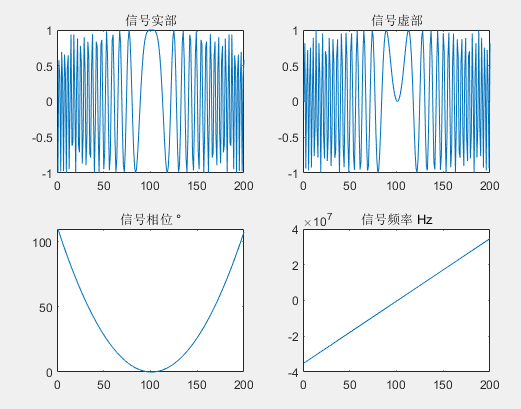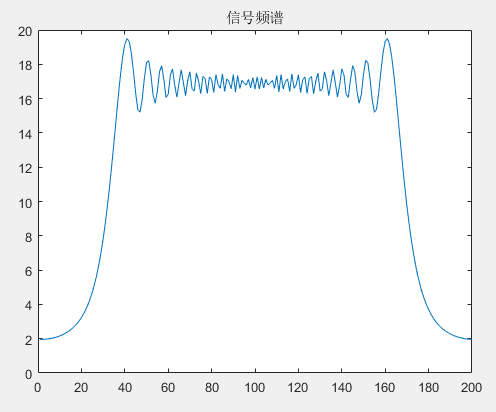01-0401-08
02-225万+
11-236765
08-319724
11-231万+
12-181163
©️2020 CSDN 皮肤主题: Age of Ai 设计师:meimeiellie